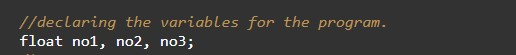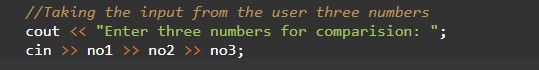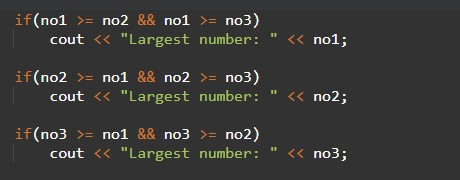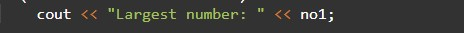# C++ Programs to Find Greatest Number

In this tutorial you will learn about the C++ Programs to Find Greatest Number and its application with practical example.

## C++ Program to Find the Greatest Number

In this tutorial, we will learn to create a C++ program that will Find Greatest Number using C++ programming.

## Prerequisites

Before starting with this tutorial we assume that you are best aware of the following C++ programming topics:

• Operators in C++ Programming.
• Basic Input and Output function in C++ Programming.
• Basic C++ programming.
• Conditional statement in C++ programming.

## Program to Find the Greatest Number

In c++ programming, it is possible to take multiple integer inputs from the user and Find the Greatest Number with the help of a very short program. The C++ language has many types of header libraries which has supported function in them with the help of these files the programming is easy. But today we will Find the Greatest Number from the taken numbers from the user using c++ programming.

Algorithm:-

With the help of this program, we can Find the Greatest Number.

## Output:-In the above program, we have first initialized the required variable.• no1 = it will hold the integer value.
• no2 = it will hold the integer value.
• no3 = it will hold the integer value.

Input numbers for the program.Program Logic Code.Printing output for the greatest number.In this tutorial we have learn about the C++ Programs to Find Greatest Number and its application with practical example. I hope you will like this tutorial.